# Trained pytorch auto-encoder model outputs junk on training subset inference

I’m trying to build a simple Auto-encoder with pytorch with the encoder being a standard convnet that outputs a latent code. The decoder is a reverse of the encoder that takes in the latent code and outputs the generated image. After a bit of training, the reconstruction is perceptually acceptable.

However, the trained model seems to generate the “right” images ONLY if the entire training dataset is passed for inference.

When I try to generate an image from the generated latent code of an existing training image, the output of the trained model is bad. But when the entire training set is passed through the model, the output for the same image is “right”.

When I pass a subset of the training set through the trained model, the output is bad but when the entire training set is passed through the trained model, the output is “right”.

Here is the auto-encoder class, model code, encoder, decoder and the results.

Can someone help me understand why this would be the case please? The model cannot be used if it works this way only. Thanks.

Auto-encoder class:

``````class AE(nn.Module):

def __init__(self,encode_seq,decode_seq):
super().__init__()
self.encoder = encode_seq
self.decoder = decode_seq

def forward(self, x):
z = self.encoder(x)
out = self.decoder(z)
return out

def sample(self, z):
return self.decoder(z)
``````

Encoder as pytorch sequential: cnnresize, View are my classes that inherit nn.Module basically to help with flattening and resizing.

``````Sequential(
(0): cnnresize(
)
(1): Conv2d(3, 128, kernel_size=(3, 3), stride=(1, 1))
(2): BatchNorm2d(128, eps=1e-05, momentum=0.1, affine=True)
(3): ReLU()
(4): Conv2d(128, 48, kernel_size=(3, 3), stride=(1, 1))
(5): BatchNorm2d(48, eps=1e-05, momentum=0.1, affine=True)
(6): ReLU()
(7): Conv2d(48, 12, kernel_size=(3, 3), stride=(1, 1))
(8): BatchNorm2d(12, eps=1e-05, momentum=0.1, affine=True)
(9): ReLU()
(10): View(
)
(11): Linear(in_features=142848, out_features=256, bias=True)
(12): BatchNorm1d(256, eps=1e-05, momentum=0.1, affine=True)
(13): ReLU()
(14): Linear(in_features=256, out_features=128, bias=True)
(15): BatchNorm1d(128, eps=1e-05, momentum=0.1, affine=True)
(16): ReLU()
(17): Linear(in_features=128, out_features=50, bias=True)
)
``````

Decoder as pytorch sequential: unView is my class that inherits nn.Module basically to help with flattening and resizing.

``````Sequential(
(0): Linear(in_features=50, out_features=128, bias=True)
(1): BatchNorm1d(128, eps=1e-05, momentum=0.1, affine=True)
(2): ReLU()
(3): Linear(in_features=128, out_features=256, bias=True)
(4): BatchNorm1d(256, eps=1e-05, momentum=0.1, affine=True)
(5): ReLU()
(6): Linear(in_features=256, out_features=142848, bias=True)
(7): unView(
)
(8): BatchNorm2d(12, eps=1e-05, momentum=0.1, affine=True)
(9): ReLU()
(10): ConvTranspose2d(12, 48, kernel_size=(3, 3), stride=(1, 1))
(11): BatchNorm2d(48, eps=1e-05, momentum=0.1, affine=True)
(12): ReLU()
(13): ConvTranspose2d(48, 128, kernel_size=(3, 3), stride=(1, 1))
(14): BatchNorm2d(128, eps=1e-05, momentum=0.1, affine=True)
(15): ReLU()
(16): ConvTranspose2d(128, 3, kernel_size=(3, 3), stride=(1, 1))
(17): View(
)
(18): Sigmoid()
)
``````

Auto-encoder class object:

``````AE(
(encoder): Sequential(
(0): cnnresize(
)
(1): Conv2d(3, 128, kernel_size=(3, 3), stride=(1, 1))
(2): BatchNorm2d(128, eps=1e-05, momentum=0.1, affine=True)
(3): ReLU()
(4): Conv2d(128, 48, kernel_size=(3, 3), stride=(1, 1))
(5): BatchNorm2d(48, eps=1e-05, momentum=0.1, affine=True)
(6): ReLU()
(7): Conv2d(48, 12, kernel_size=(3, 3), stride=(1, 1))
(8): BatchNorm2d(12, eps=1e-05, momentum=0.1, affine=True)
(9): ReLU()
(10): View(
)
(11): Linear(in_features=142848, out_features=256, bias=True)
(12): BatchNorm1d(256, eps=1e-05, momentum=0.1, affine=True)
(13): ReLU()
(14): Linear(in_features=256, out_features=128, bias=True)
(15): BatchNorm1d(128, eps=1e-05, momentum=0.1, affine=True)
(16): ReLU()
(17): Linear(in_features=128, out_features=50, bias=True)
)
(decoder): Sequential(
(0): Linear(in_features=50, out_features=128, bias=True)
(1): BatchNorm1d(128, eps=1e-05, momentum=0.1, affine=True)
(2): ReLU()
(3): Linear(in_features=128, out_features=256, bias=True)
(4): BatchNorm1d(256, eps=1e-05, momentum=0.1, affine=True)
(5): ReLU()
(6): Linear(in_features=256, out_features=142848, bias=True)
(7): unView(
)
(8): BatchNorm2d(12, eps=1e-05, momentum=0.1, affine=True)
(9): ReLU()
(10): ConvTranspose2d(12, 48, kernel_size=(3, 3), stride=(1, 1))
(11): BatchNorm2d(48, eps=1e-05, momentum=0.1, affine=True)
(12): ReLU()
(13): ConvTranspose2d(48, 128, kernel_size=(3, 3), stride=(1, 1))
(14): BatchNorm2d(128, eps=1e-05, momentum=0.1, affine=True)
(15): ReLU()
(16): ConvTranspose2d(128, 3, kernel_size=(3, 3), stride=(1, 1))
(17): View(
)
(18): Sigmoid()
)
)
``````

Model that I use to run epochs: Input to the model is in shape (no_examples,channels,height,width)

``````# MAIN AE MODEL
# --------------

def model_ae(x,epochs,mbsize,net,lr_rate):

global rc_loss #For plotting costs
rc_loss = []

# Setting up minibatch features
m = x.size()
mb_list = []
mb_list = list(range(int(m/mbsize)))
if m % mbsize == 0: # if the minibatches can be split up perfectly.
'do nothing'
else:
mb_list.append(mb_list[len(mb_list)-1] + 1)

for i in range(epochs):
for p in mb_list:

# Mini batch operations
start_index = p*mbsize
end_index = m if p == mb_list[len(mb_list)-1] else p*mbsize + mbsize

# we now have the start and end indices for X.
X_mb = x[start_index:end_index]
m_curr = end_index - start_index

images = X_mb.view(X_mb.size(0), -1)# Flattening images
out = net(images)

# Compute reconstruction loss
reconst_loss = F.binary_cross_entropy(out, images)

# Backprop + Optimize
rc_loss_example_k_pixels = reconst_loss.data # for printing only
rc_loss.append(rc_loss_example_k_pixels) # for plotting only

print('Epoch ' + str(i+1) + ', minibatch ' + str(p+1) + ' of '  +  str(len(mb_list)) +', RC loss per example / k pixels: ' + str(rc_loss_example_k_pixels))
reconst_loss.backward()
optimizer.step()
``````

Finally, here is the output of the trained model. Training set is of size - torch.Size([82, 3, 130, 102]). The function I use to sample images is also give below:

``````def sampleimages(x,net,c,h,w):
out_image = net(x)
try:
out_image = out_image.cpu()
except:
'do nothing'
out_image = out_image.view(out_image.size(),c,h,w)
out_image = out_image.data.numpy()
out_image = np.swapaxes(out_image,1,3)
out_image = np.swapaxes(out_image,1,2)
return out_image
``````
1. Output on the entire training set xtrain of size [82, 3, 130, 102]:
``````done_image = sampleimages(xtrain,ae,3,130,102)
for i in range(0,2):
plt.imshow(done_image[i])
plt.show()
``````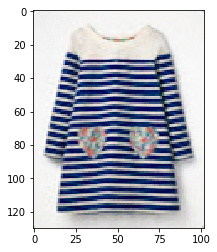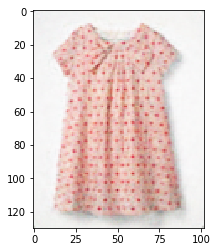1. Output on subset xtrain[0:5] of size[5, 3, 130, 102]: The two images below belong to the same indices as above images.
``````done_image = sampleimages(xtrain[0:5],ae,3,130,102)
for i in range(0,2):
plt.imshow(done_image[i])
plt.show()
``````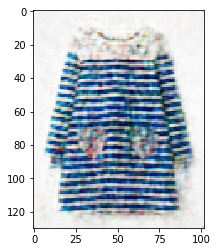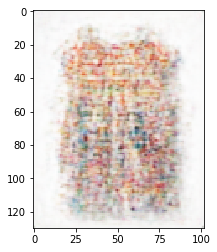1. Interesting as I increase the subset size to round 20, the quality of the output gets better. Output on subset xtrain[0:20] of size[20, 3, 130, 102]: The two images below belong to the same indices as above images.
``````done_image = sampleimages(xtrain[0:20],ae,3,130,102)
for i in range(0,2):
plt.imshow(done_image[i])
plt.show()
``````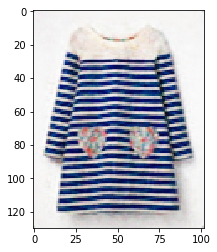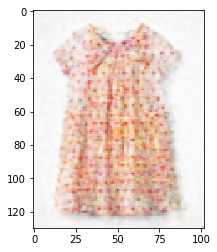Any help would be great, thanks in advance.

I guess you didn’t set your model to evaluation before generating the samples. Since you are using some `BatchNorm` layers, the running statistics will be calculated and updated using the current batch. Try to call `model.eval` before the inference step. This will make sure to use the approximate running mean and var.
Sometimes it might help to pass some validation inputs in training mode without backpropagating to update the `BatchNorm` layers.

Let me know, if my assumption was right.

1 Like

Absolutely right. I knocked off the BT layers and ran the model from scratch again and it ran well on single image latent z’s as well. Will use model.eval from inference step going forward